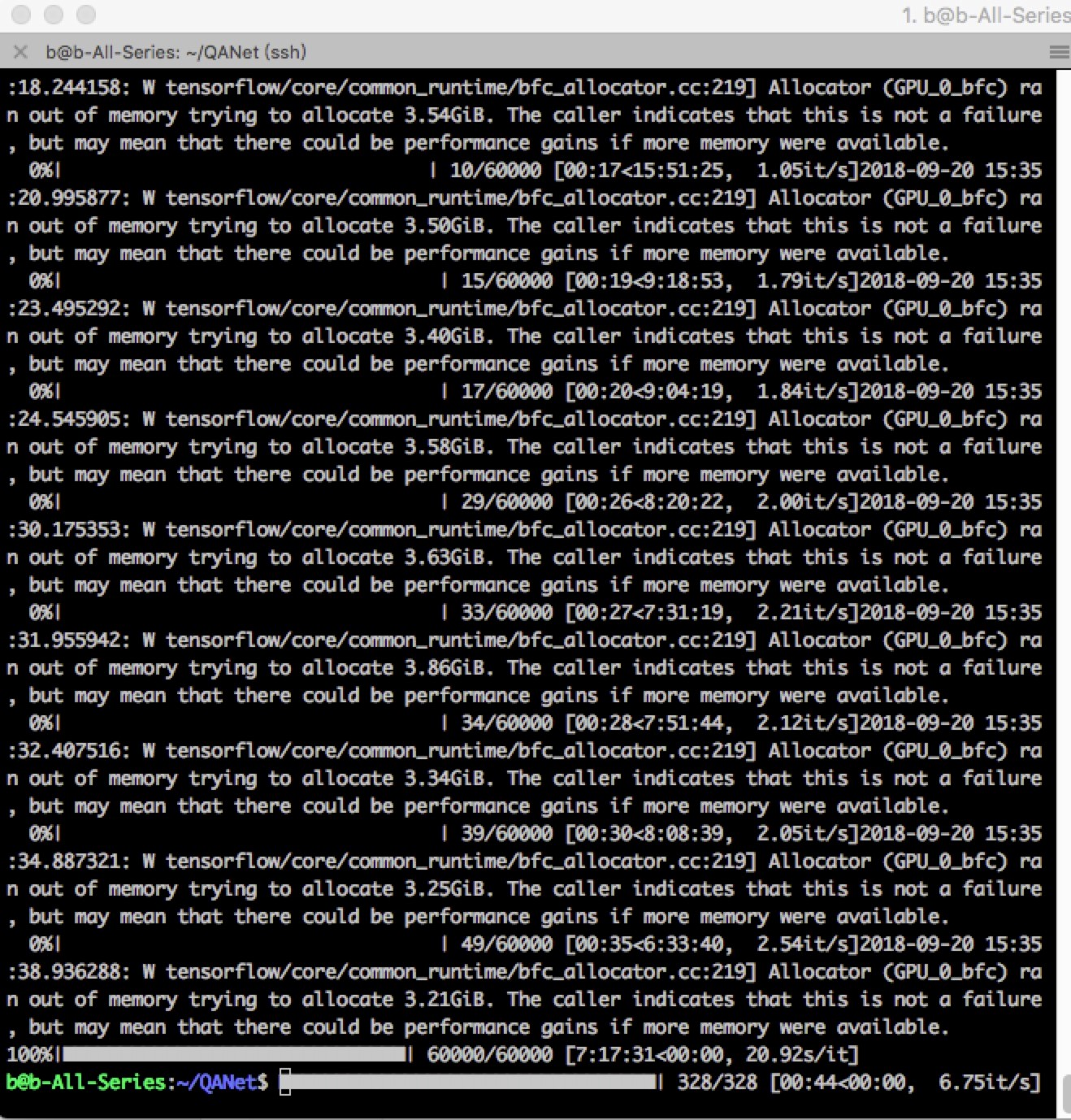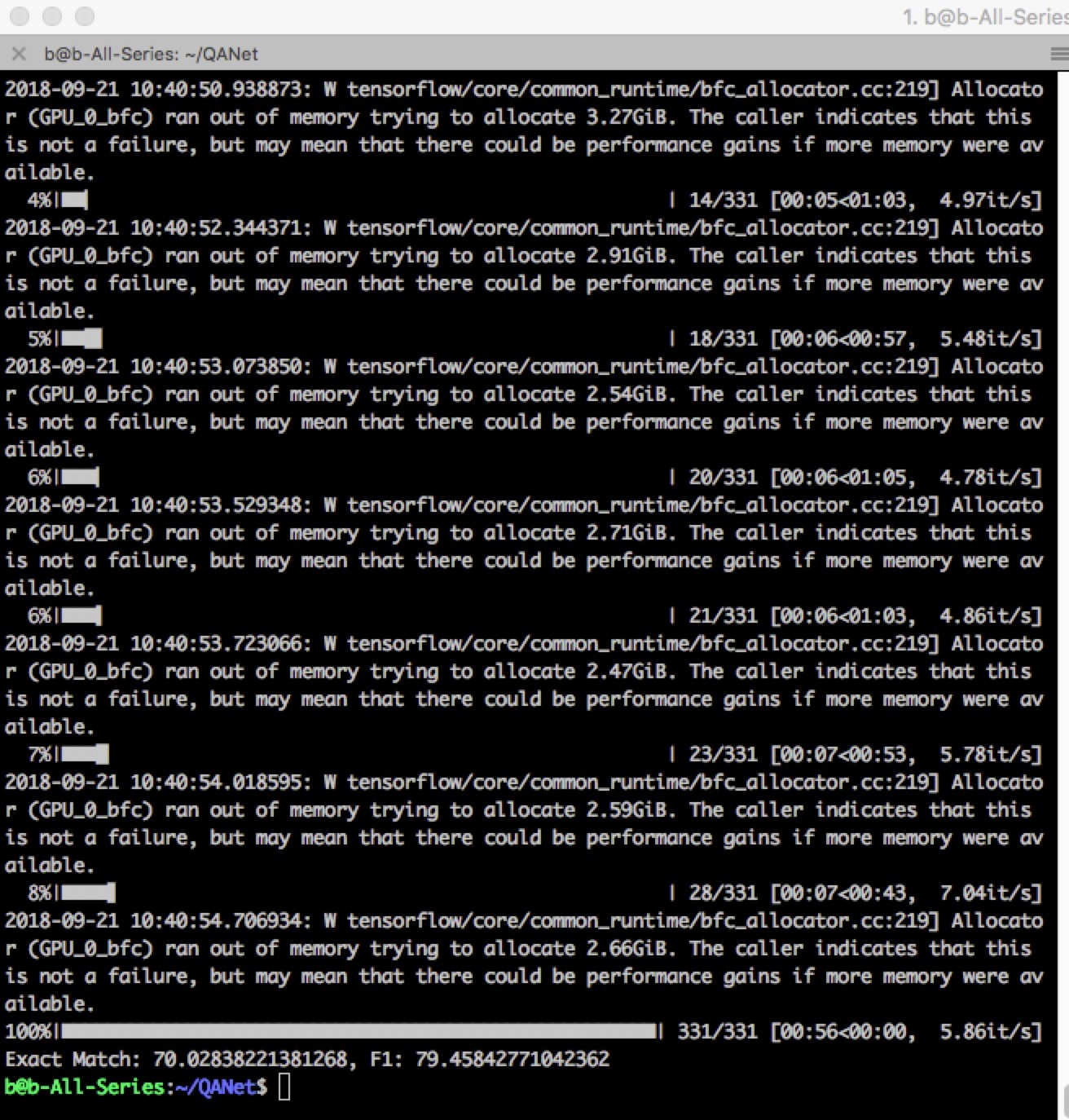# 用卷积神经网络和自注意力机制实现QANet（问答网络）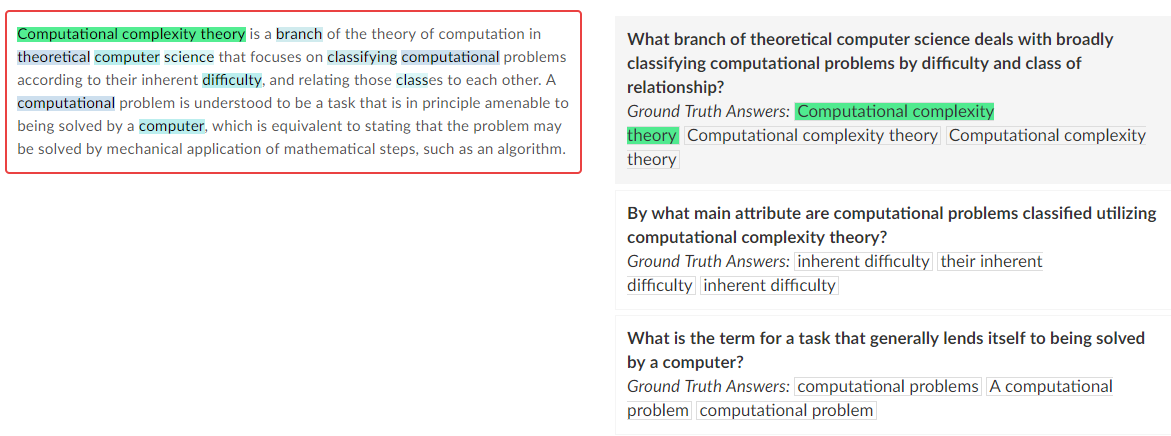P: “Computational complexity theory is a branch of the theory of computation in theoretical computer science that focuses on classifying computational problems according to their inherent difficulty, and relating those classes to each other. A computational problem is understood to be a task that is in principle amenable to being solved by a computer, which is equivalent to stating that the problem may be solved by mechanical application of mathematical steps, such as an algorithm.”

Q: “What is the term for a task that generally lends itself to being solved by a computer?”

A: “computational problem”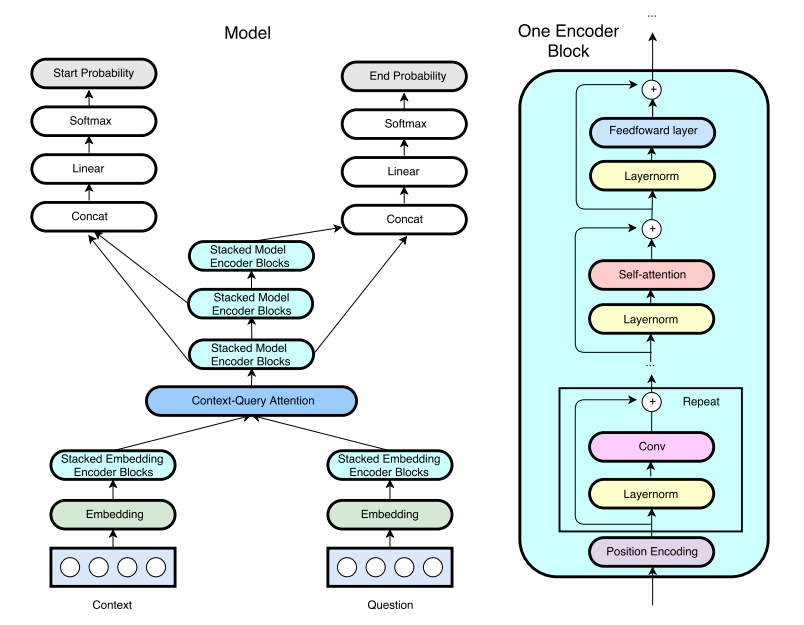1.词嵌入

2.编码器

3.注意力机制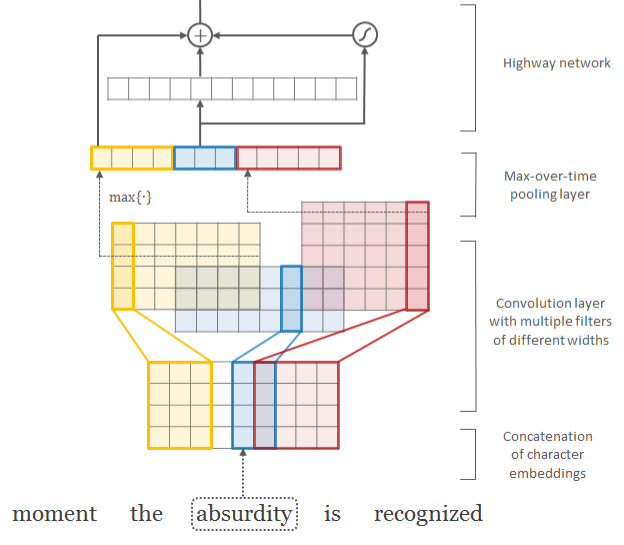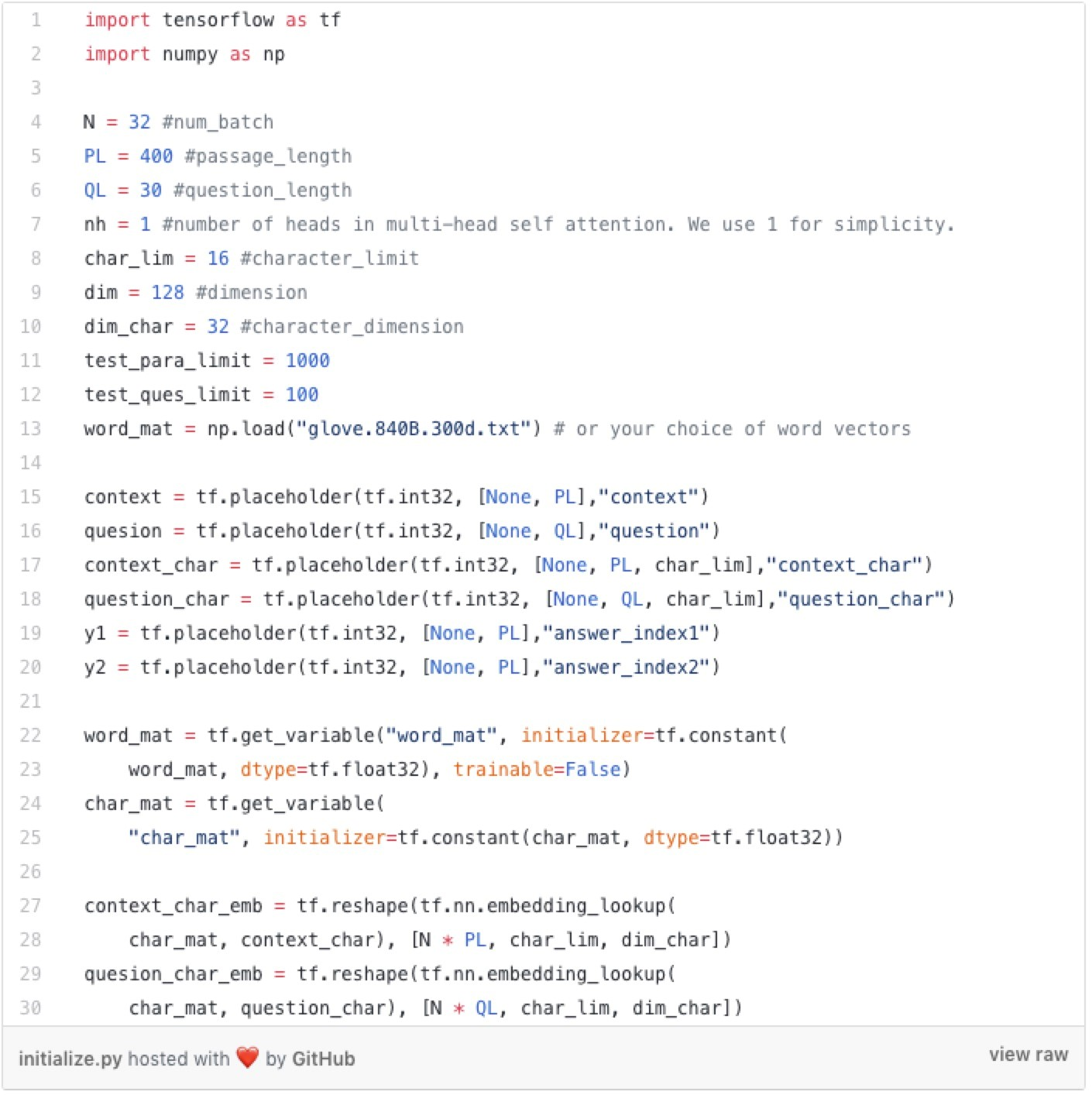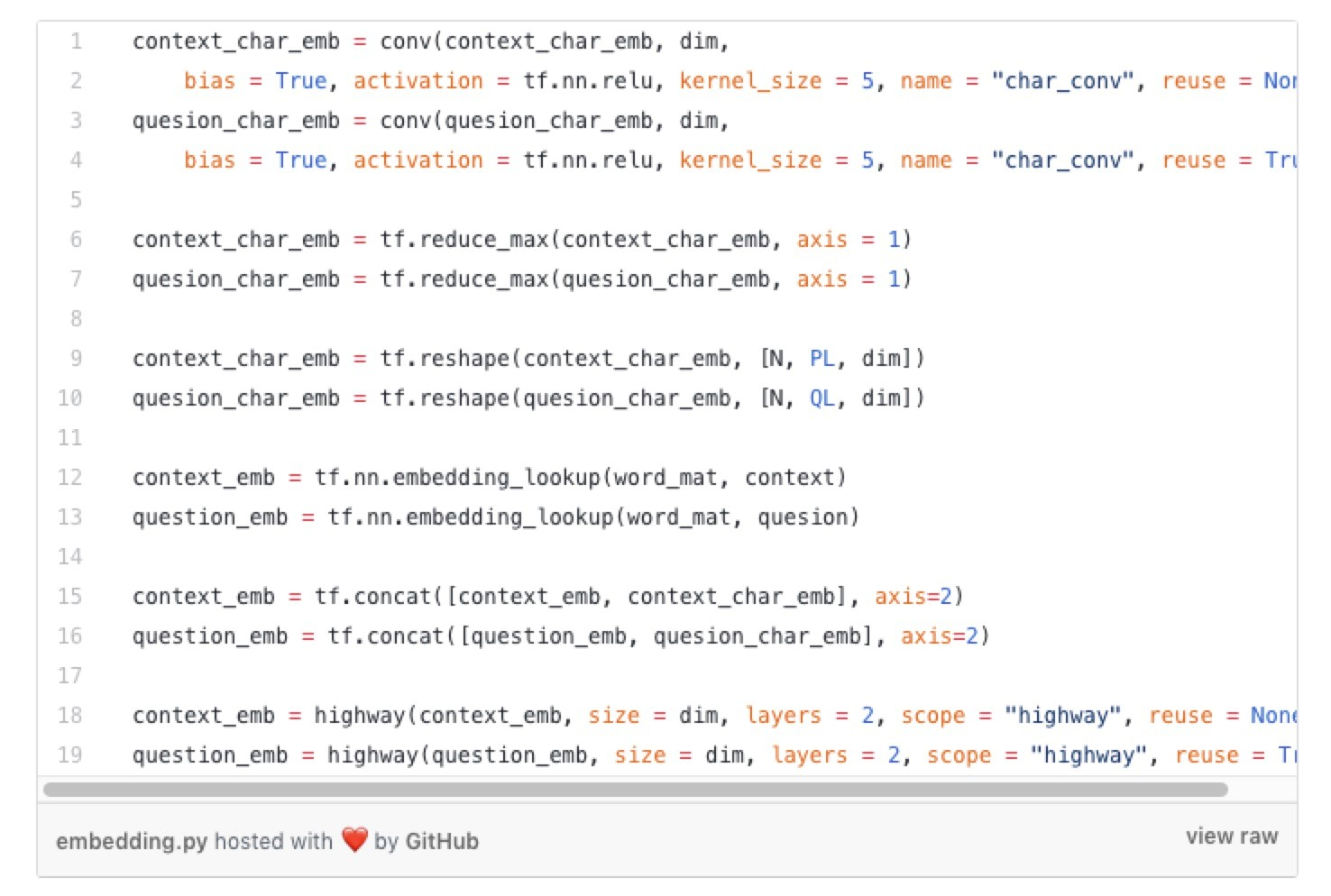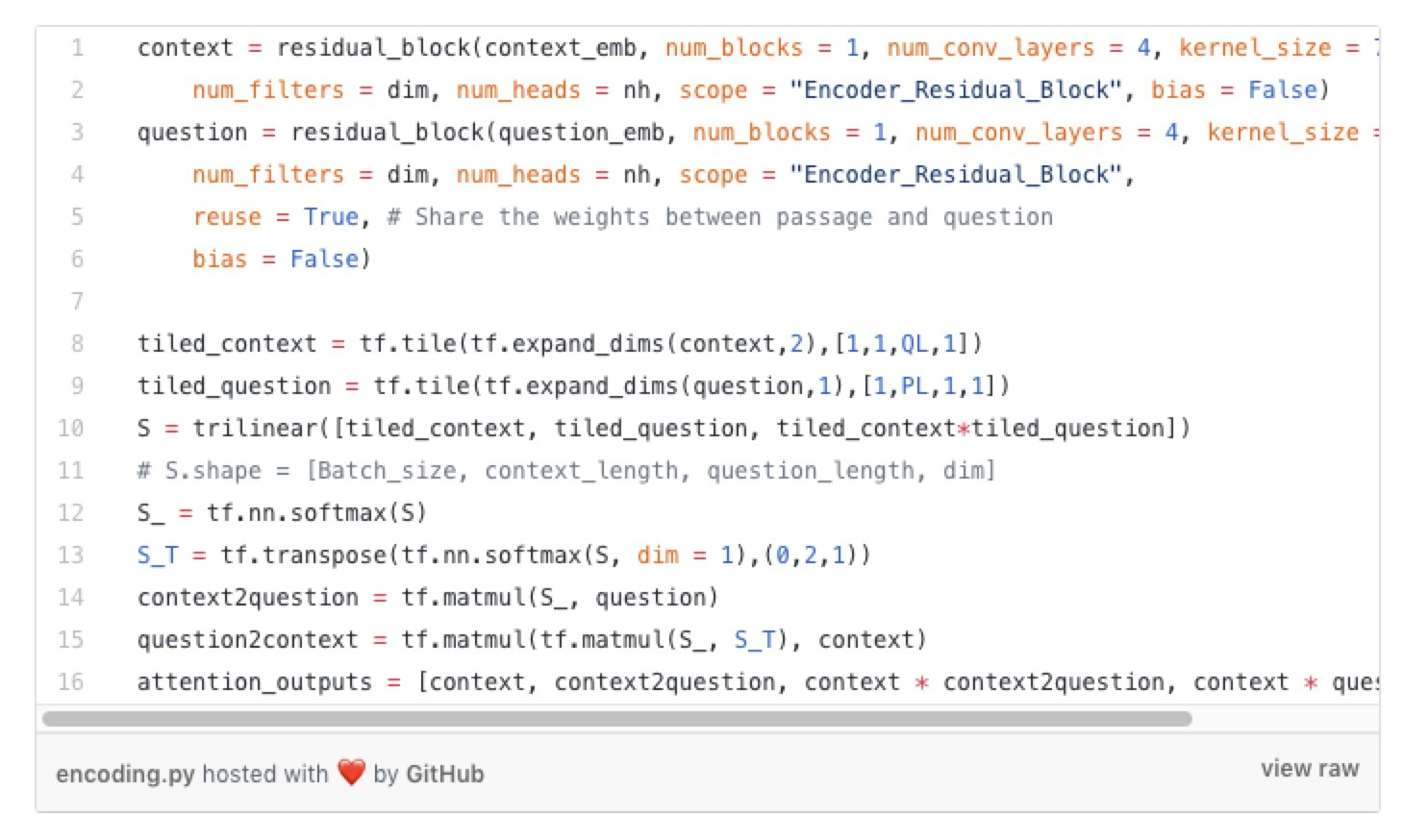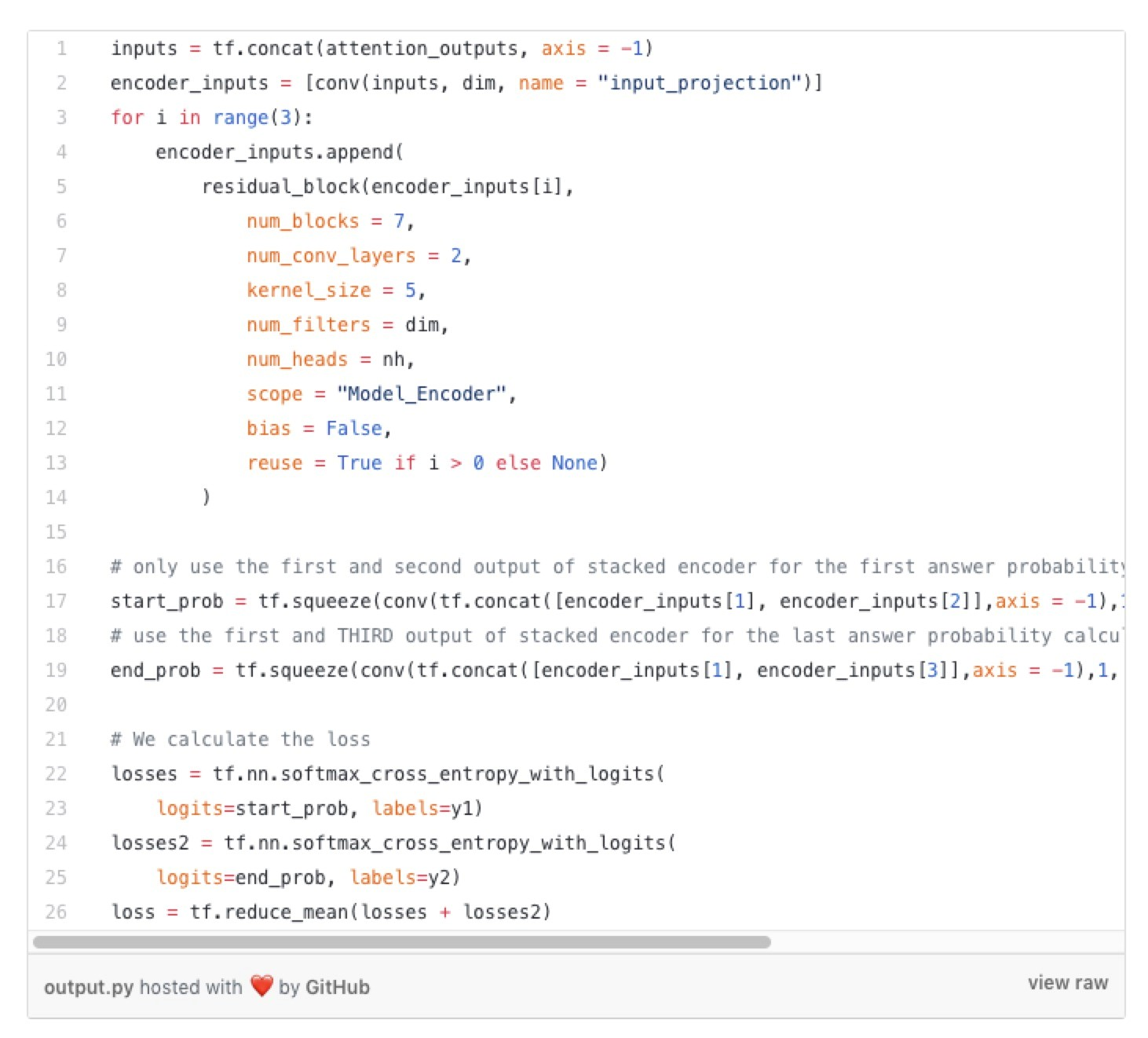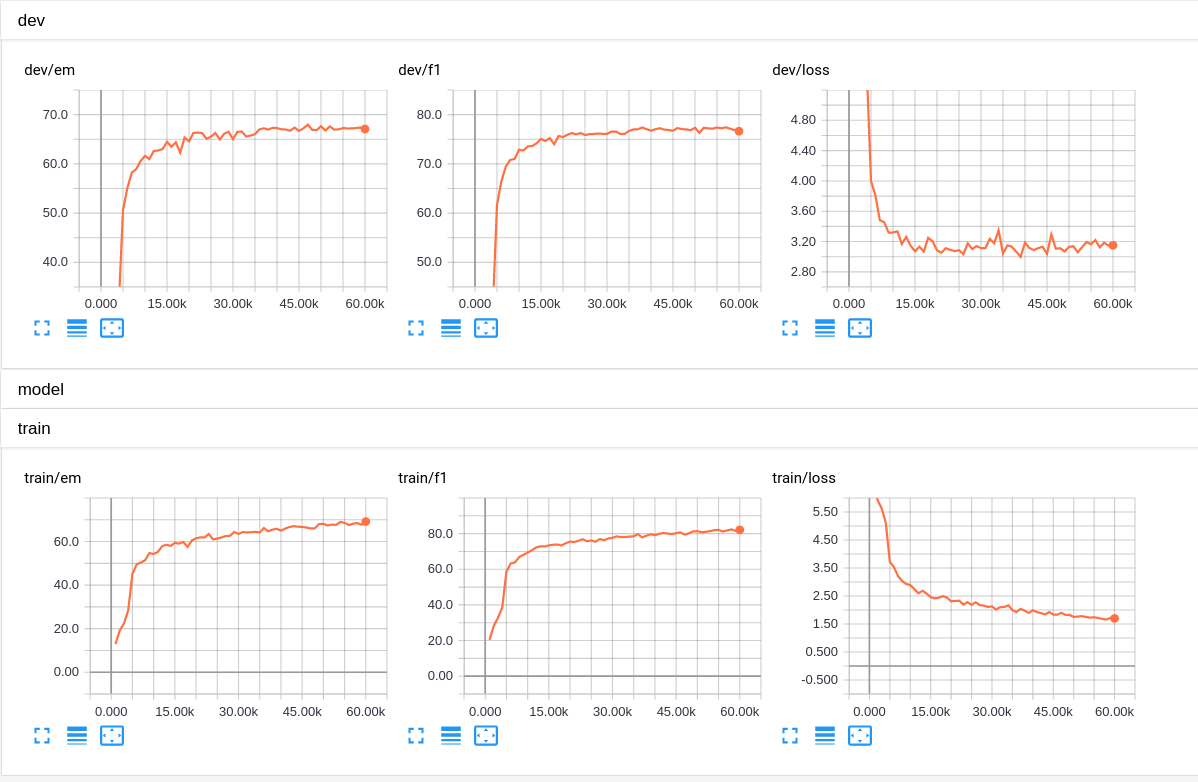https://github.com/NLPLearn/QANet